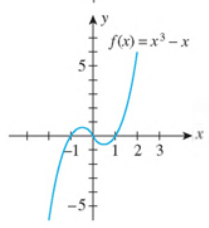Chapter 2.4, Problem 64E

Chapter
Section
Textbook Problem

In Exercises 63-68, use the graph of the function f to determine lim x → ∞ f ( x ) and lim x → − ∞ f ( x ) 64.To determine
The limit of given expression with the help of the given graph.

Explanation

The given graph of the function f(x)=x3x is shown below.

Figure (1)

It is clearly observed from the above graph of f(x) that as the value of x approaches to infinity the value of the function f(x)=x3x also approaches to infinity

Still sussing out bartleby?

Check out a sample textbook solution.

See a sample solution

The Solution to Your Study Problems

Bartleby provides explanations to thousands of textbook problems written by our experts, many with advanced degrees!

Get Started

Evaluate limt0t3tan3(2t)

Single Variable Calculus: Early Transcendentals, Volume I

In Problems 7-34, perform the indicated operations and simplify. 30.

Mathematical Applications for the Management, Life, and Social Sciences

Find f'(a). f(t)=2t+1t+3

Single Variable Calculus: Early Transcendentals

Solve each equation: x2x=42

Elementary Technical Mathematics

f(x) = x3 2x2 + x 1 has a local maximum at: a) 0 b) 1 c) 13 d) f has no local maxima

Study Guide for Stewart's Single Variable Calculus: Early Transcendentals, 8th